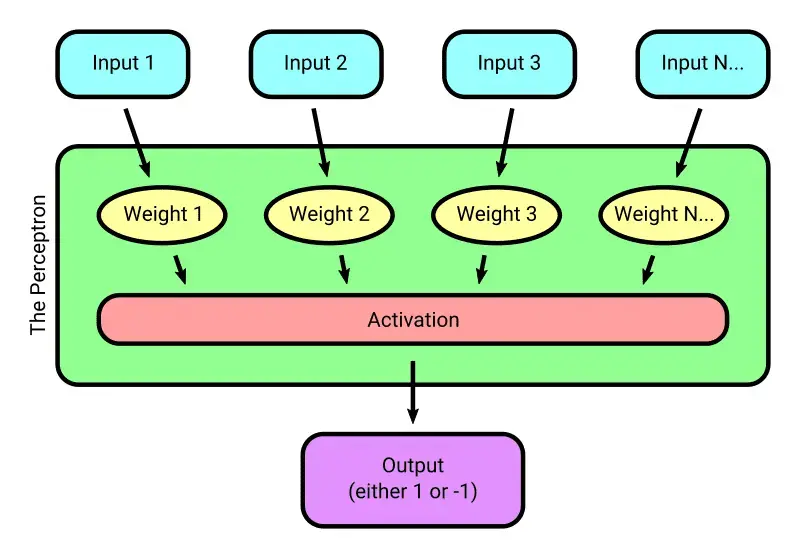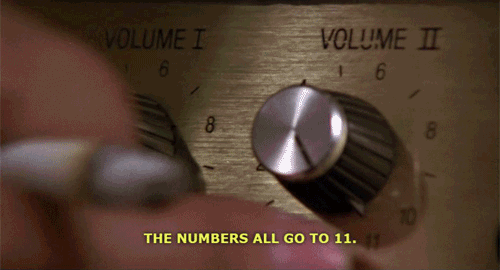Insights

# The humble perceptron

Maybe you have heard the term “neural network” and possibly you have a basic understanding of what it means. It’s a kind of software that receives input and “learns” how to produce correct output. But, if you are anything like I was not too long ago, what it means for the neural network to “learn” is a complete black box. Input goes in, magic happens, good things come out

That was the extent of my understanding until recently, when I decided to fill the gaps and develop a basic working knowledge of neural networks. I wanted to know what was happening in that “magic” step. How exactly did this software “learn?”

This article represents my first step in that pursuit. I focused on one concept in particular: the perceptron

What is a perceptron? And why start there? A perceptron is a binary classifier, which means it can only return one of two possible outputs (“classifications”). A simple way to think about it is that a perceptron is useful for answering “yes” or “no” kinds of questions like, “Do you want a cup of coffee?”

But the really cool thing about perceptrons — and the reason it is a perfect place to start learning about neural networks — is that a perceptron is a basic implementation of a single neuron. A “neural network” is simply a group of connected neurons. If we can understand how a single neuron works, understanding how they all work together in a network is surprisingly intuitive

Before we get into it, a disclaimer: I am no expert in the fields of data science or machine learning. I am writing this because I found that understanding the perceptron was that lightbulb moment I needed to peer into the magic black box of neural networks. Once I understood this basic building block, the rest started to fall into place. I still have so much to learn, but now I have a fundamental understanding from which to build. I hope by the end of this article you will, too!

## The anatomy of a perceptron

First let’s define some terms and talk about the components of a perceptron. Use this diagram to orient yourself as we walk through each component:## Input

The input of a perceptron is the numeric data fed into the perceptron for which we want a binary classification. For example, if you were using a perceptron to determine whether or not you’d like a cup of coffee, you might have the following numeric input:

• Do you like coffee? (1 or 0)
• Time of day in minutes (0 to 1440)
• Your tolerance for caffeine (between 1 and 10)
• How tired are you? (between 1 and 10)
• How much effort obtaining a cup of coffee will require (between 1 and 10)

Now, given a dataset like above, a perceptron should be able to learn and then somewhat accurately predict whether or not you’d like a coffee. But how? You’ll just have to… “weight”… to find out!

### Weight

It’s perhaps a little simplistic, but you could think of “weight” as the amount of impact a particular input has on the outcome. For example, if you really like coffee, you may be willing to drink it any time of day! In that case, the weight associated with “Do you like coffee” would be very high and the weight associated with “Time of day in minutes” would be low

Each input has its own weight. When input is passed into the perceptron, each input is multiplied by its corresponding weight. The resulting value is then added together with all the other input/weight combinations, and that single number is what is passed to the “activation” function

### Activation

The “activation” function determines whether the neuron is active or inactive. It takes the sum of all the inputs multiplied by their corresponding weights (an aggregate weighted input) and converts it to the expected binary output. This can get complicated and involve other concepts like “bias,” but a simple example of an activation function would be something like: If our aggregate input is greater than 0, return 1. Otherwise, return -1

### Output

The output of a perceptron is simply the result of the activation function. It can only ever be one of the two values representing either “active” or “inactive.” In this article I’ve used 1 for active and -1 for inactive.

## Training a perceptron

That’s all well and good, but how does a perceptron “learn?” A perceptron “learns” through a process called “supervised learning” or “training.” Here’s how it works: First, you need a large set of example inputs (training data) for which you already know the correct output (active/inactive). Here’s an example using the coffee dataset from before:

Output: 1. Yes, I’ll absolutely have a coffee at 8 p.m., please.

Once you’ve collected a good number of inputs with corresponding correct outputs as seen above, you’re ready to train your perceptron! You train it by running each set of inputs through the perceptron one at a time. If the perceptron gets it wrong, that information is used to make changes to the weights associated with the inputs. The idea is that after those adjustments, the perceptron is more likely to produce correct output when that dataset (or datasets similar to it) get passed into the perceptron in the future

So, training is actually simple — it’s just adjusting weights. You could think of those weights as knobs. When you turn each one to the optimal number, the perceptron will be most likely to produce the correct output for any inputBut how do you know how to adjust the weights? Each weight is adjusted individually with a function that takes into account the individual input corresponding to the weight, the current weight value, and the output. There are some fancier things you can do, but the basic function looks something like this:

newWeight = currentWeight + (correctAnswer – incorrectGuess) * input

## An example

Are you the kind of person that has to see code and get your hands dirty to learn? Me too. While I was learning, I put together this example perceptron implementation with a visualization of its training process:The problem I’m using the perceptron to solve is very simple: Given two inputs that represent the coordinates of a point on a two-dimensional plane (x and y), determine whether that point is in the upper right half of the plane (represented by blue) or the lower left half (represented by yellow). The black line is there to visualize the truth threshold between the two halves of the plane, while the purple line visualizes the perceptron’s current “understanding” of the threshold location

Each time you click “Train Again,” a new dot is added to the plane at random and the perceptron guesses whether the dot should be yellow or blue. You’ll notice in the image above the perceptron got it wrong a few times while it was learning! When the perceptron gets it wrong, we feed that back into the training function and update its weights. This results in the purple line moving to visualize the perceptron’s new “understanding.”

Eventually the perceptron’s weights are adjusted to the point that it essentially always gets it right. When we reach this state, the purple line will be in almost the exact same position as the black line

Of course this is a silly example. You could solve this problem programmatically without any machine learning whatsoever in just one line of code. But shh! Our perceptron doesn’t know that. Let it learn! Try it out! Tweak the code and see what happens to the learning process!

## Putting it together: The network!

A single perceptron on its own is a surprisingly effective tool, but it is limited to binary classification (“yes” or “no” kinds of questions). But what if we had multiple perceptrons? And what if the output of a set of perceptrons served as the input for another set of perceptrons? Now you have a network of neurons — you might even call it a “neural network!”A network like this could be configured in a number of different ways to solve all sorts of complex problems. For example, you could change the number of layers and the number of neurons each layer contains. Training a neural network looks much the same as training an individual perceptron: Feed training data in, when the output is incorrect, adjust the weights within each individual perceptron.

## Conclusion

Of course there is much more to neural networks than what we’ve covered here, but I want to demonstrate that the fundamental concept is quite simple. If you’ve understood the perceptron (a single neuron) then a neural network is essentially just layers of perceptrons feeding into one another

If you’re interested in learning more about machine learning and neural networks, I recommend this video series by Daniel Shiffman — from which I learned much of what I’ve shared in this article. It’s very beginner-friendly and thorough

Happy (machine) learning!